# 100 100 200 200 300 300 400 400

• Slides: 38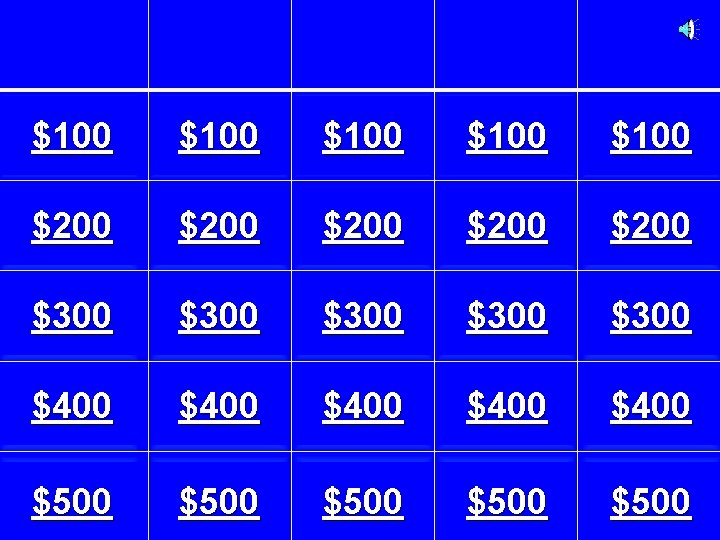\$100 \$100 \$200 \$200 \$300 \$300 \$400 \$400 \$500 \$500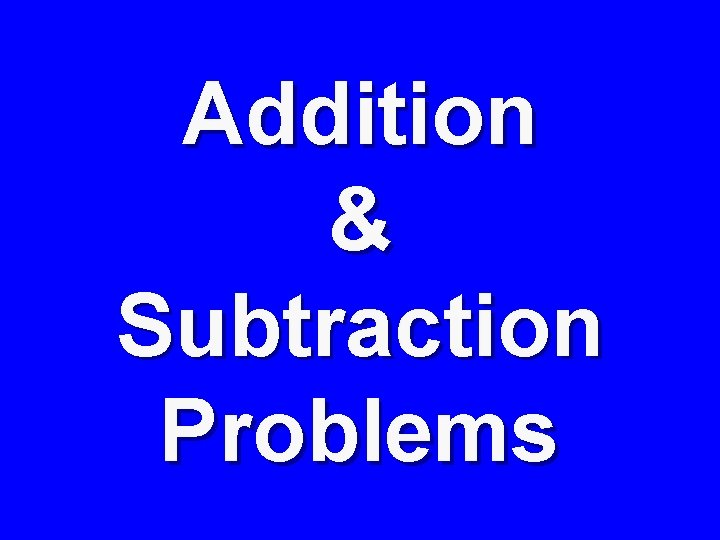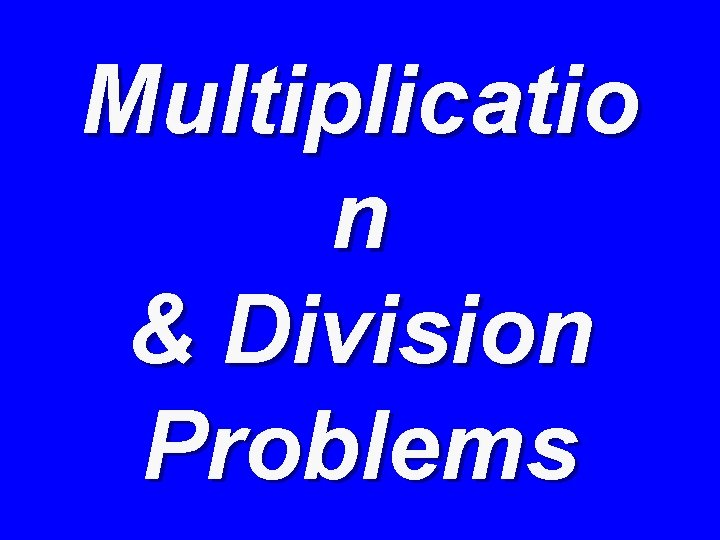Multiplicatio n & Division ProblemsWriting Expression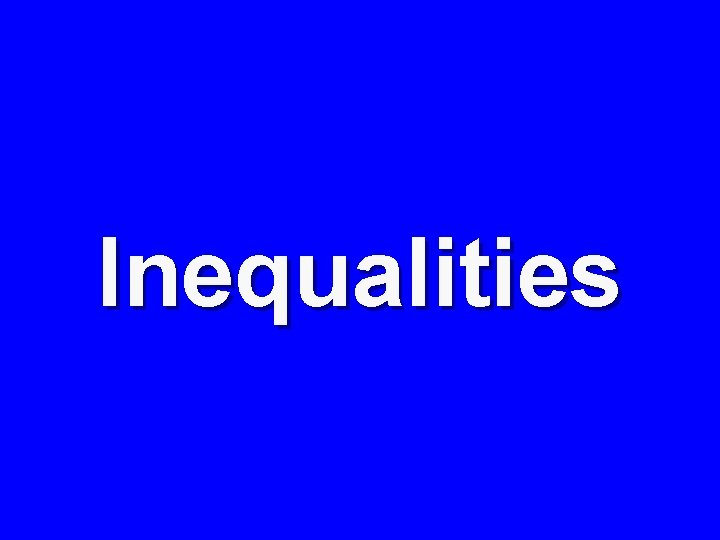Inequalities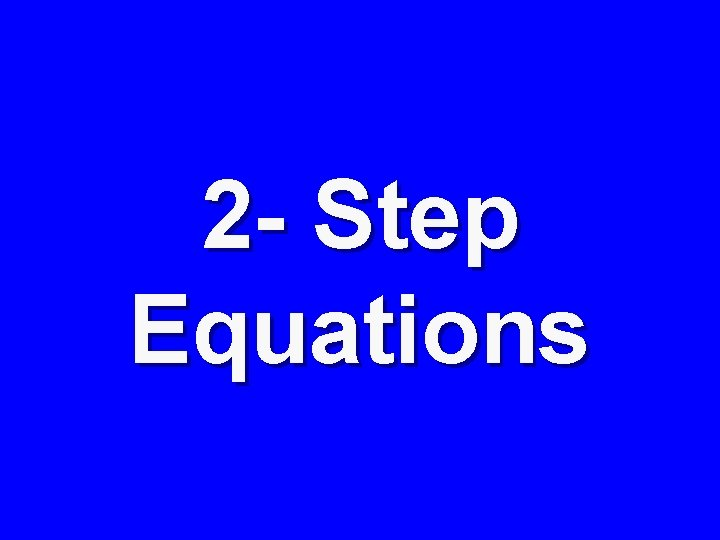2 - Step Equations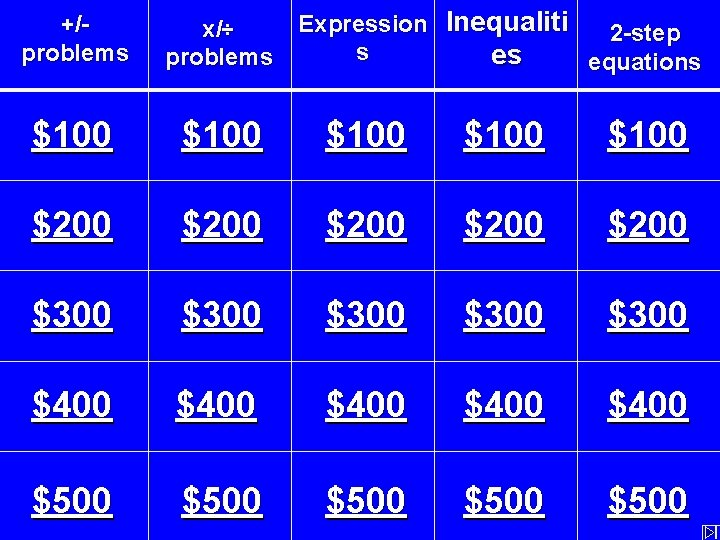Expression Inequaliti 2 -step s es equations +/problems x/÷ problems \$100 \$100 \$200 \$200 \$300 \$300 \$400 \$400 \$500 \$500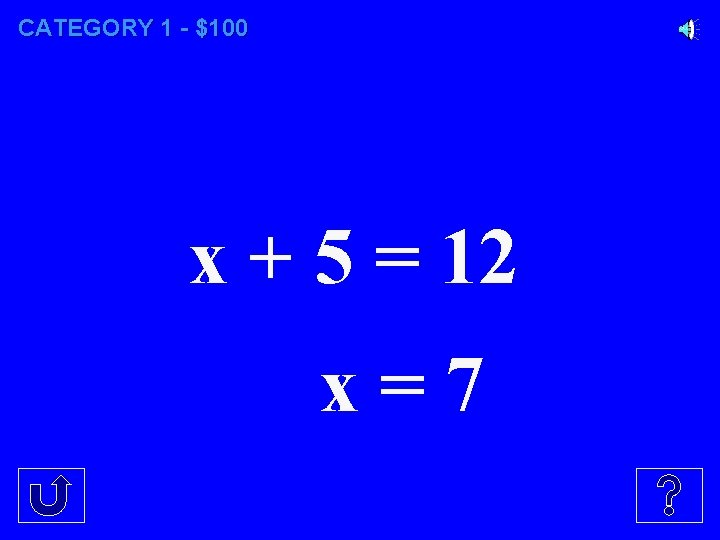CATEGORY 1 - \$100 x + 5 = 12 x=7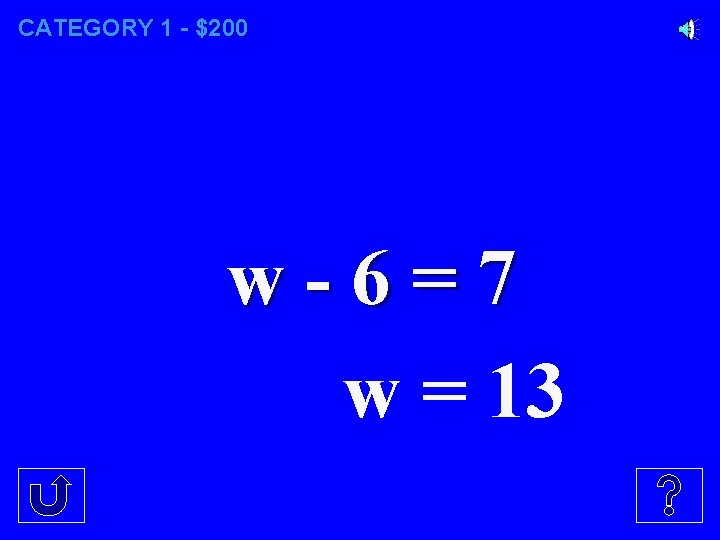CATEGORY 1 - \$200 w-6=7 w = 13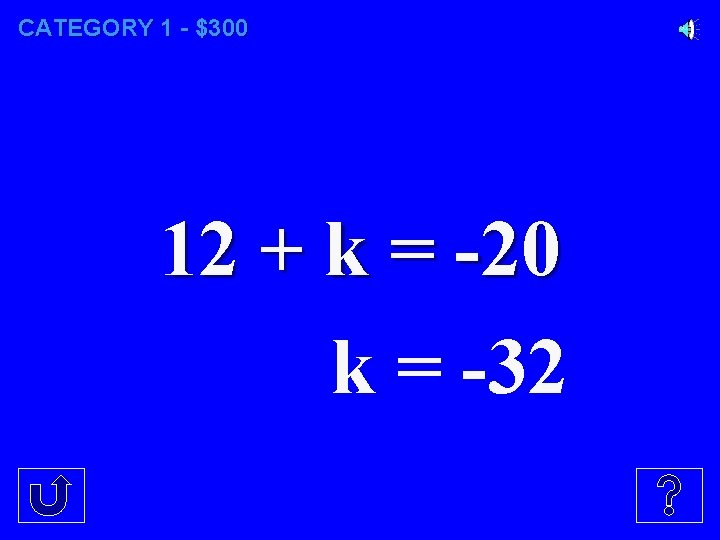CATEGORY 1 - \$300 12 + k = -20 k = -32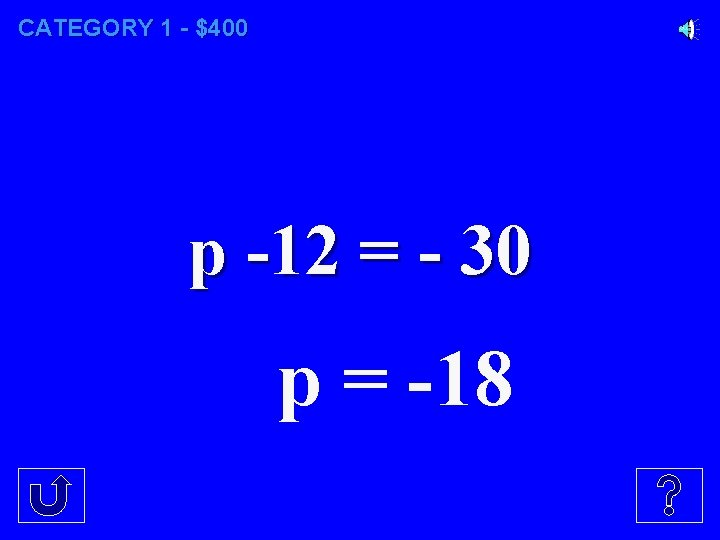CATEGORY 1 - \$400 p -12 = - 30 p = -18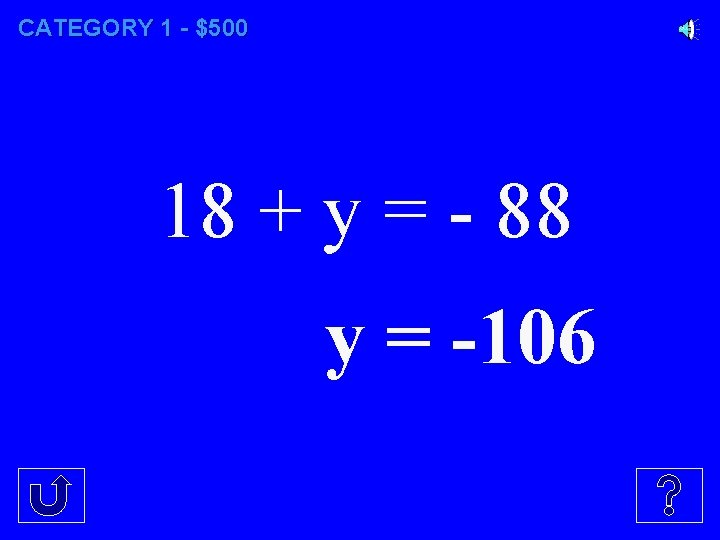CATEGORY 1 - \$500 18 + y = - 88 y = -106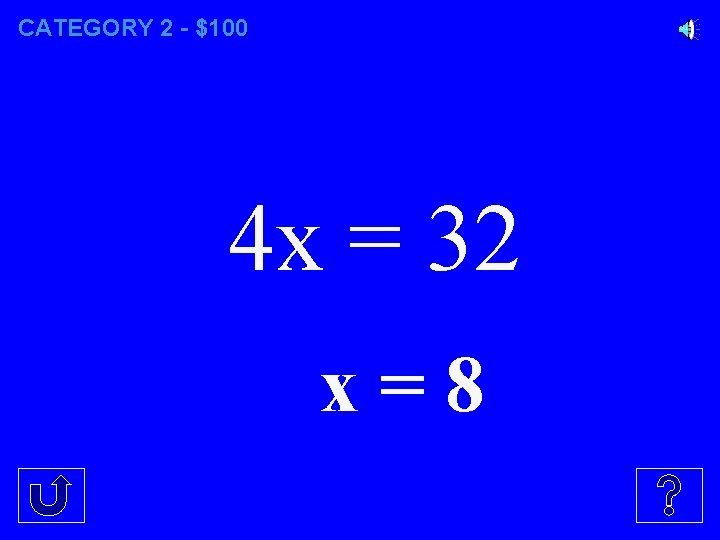CATEGORY 2 - \$100 4 x = 32 x=8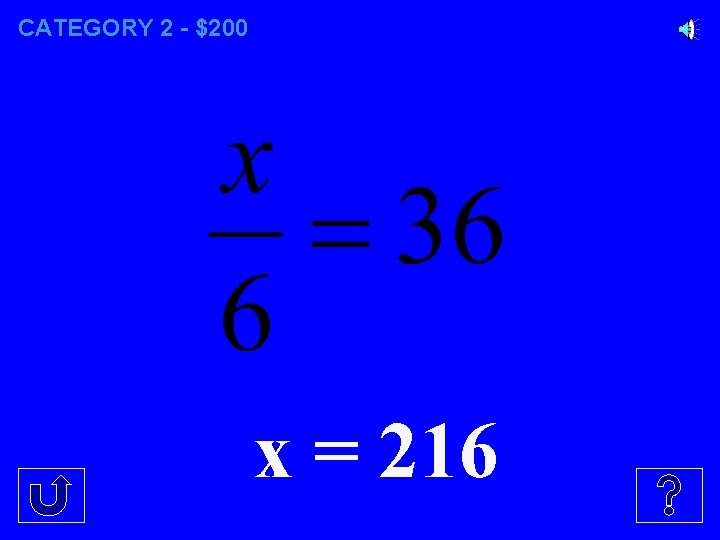CATEGORY 2 - \$200 x = 216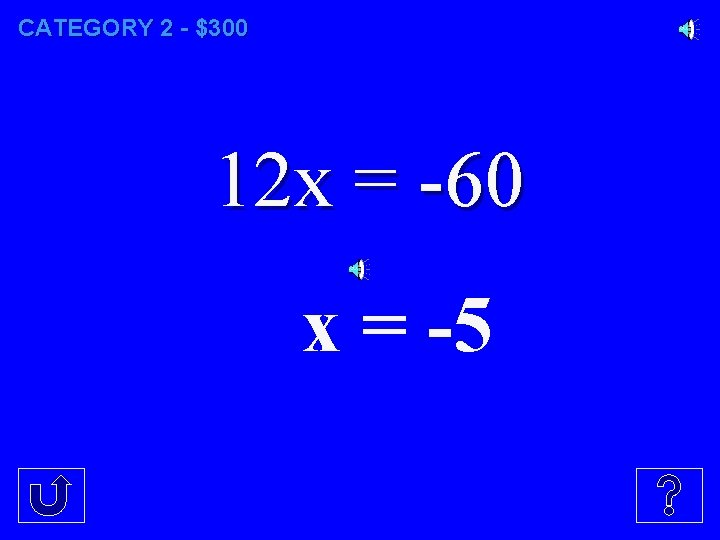CATEGORY 2 - \$300 12 x = -60 x = -5CATEGORY 2 - \$400 x = -36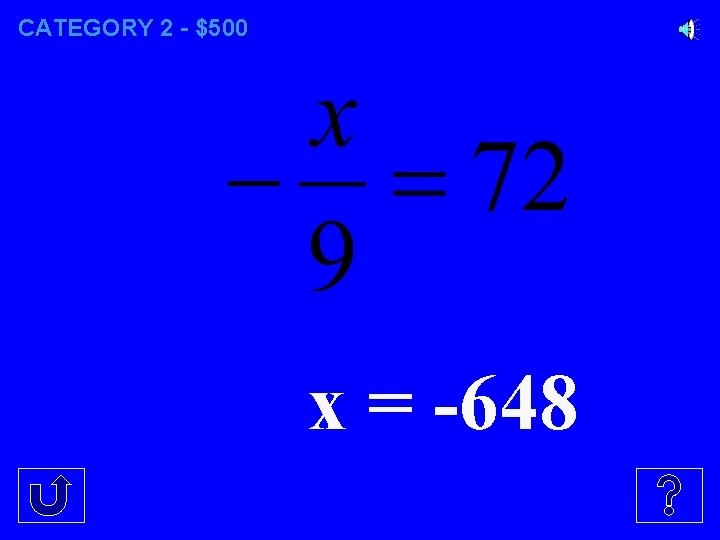CATEGORY 2 - \$500 x = -648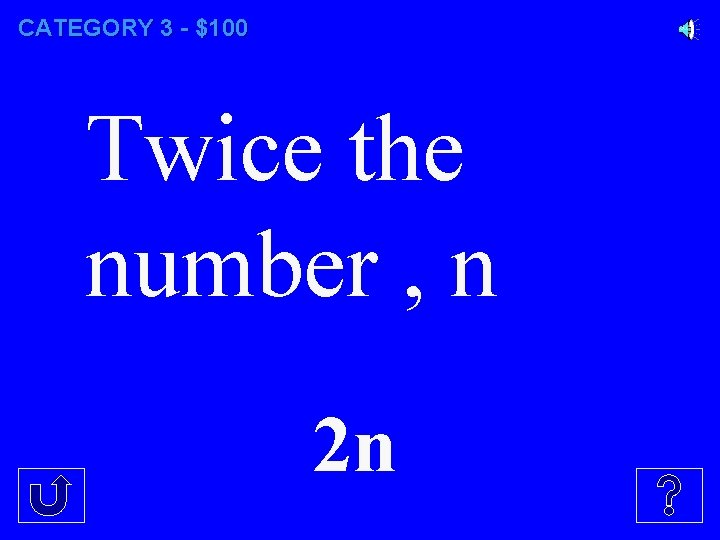CATEGORY 3 - \$100 Twice the number , n 2 nCATEGORY 3 - \$200 five less than the number, q q-5CATEGORY 3 - \$300 The difference of w and 4 w-4CATEGORY 3 - \$400 \$220 per day plus \$10 220 d + 10CATEGORY 3 - \$500 1/3 the sum of a number x and 7 1/3( x + 7)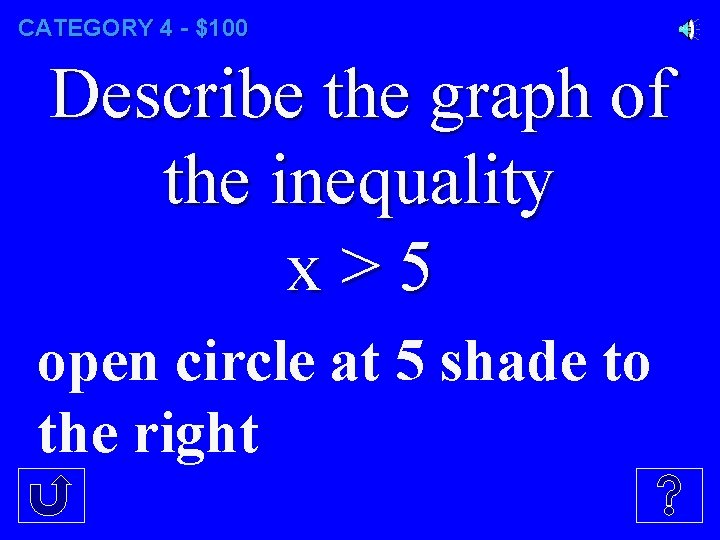CATEGORY 4 - \$100 Describe the graph of the inequality x>5 open circle at 5 shade to the rightCATEGORY 4 - \$200 Is 2 a solution to 6 ≤ 3 x +1 yes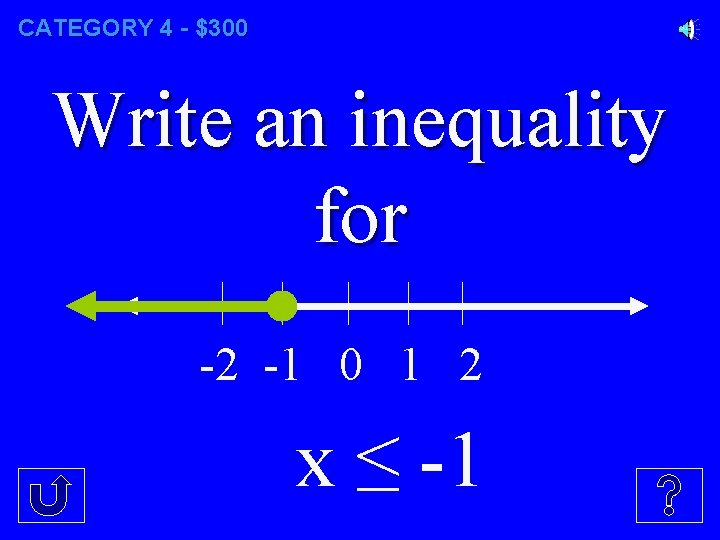CATEGORY 4 - \$300 Write an inequality for -2 -1 0 1 2 x ≤ -1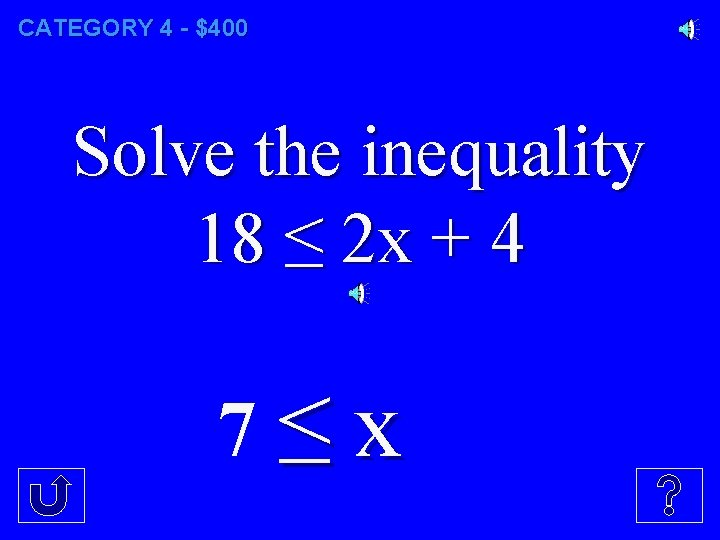CATEGORY 4 - \$400 Solve the inequality 18 ≤ 2 x + 4 7≤x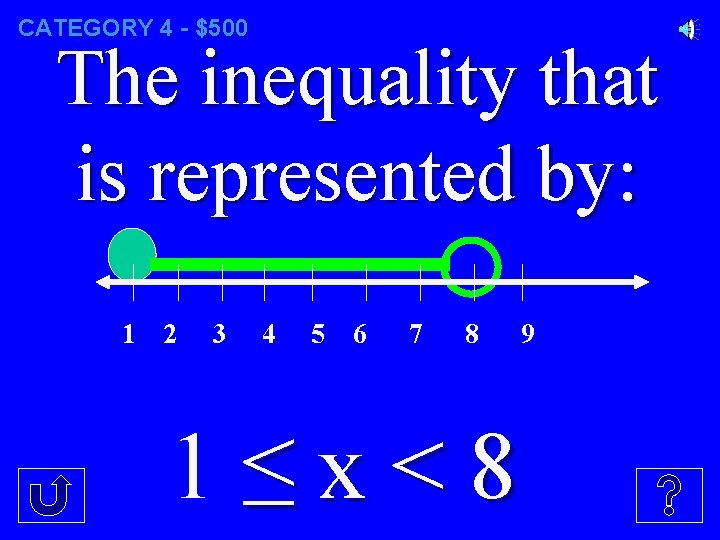CATEGORY 4 - \$500 The inequality that is represented by: 1 2 3 4 5 6 7 8 1≤x<8 9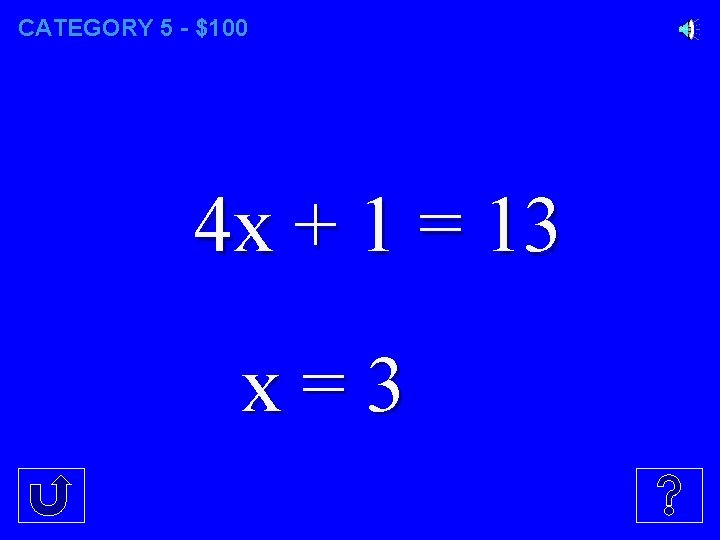CATEGORY 5 - \$100 4 x + 1 = 13 x=3CATEGORY 5 - \$200 -2 x – 5 = 25 x = -15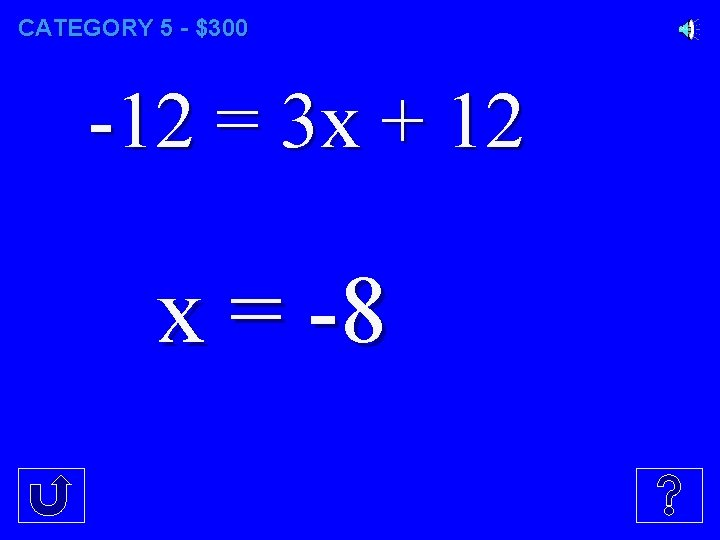CATEGORY 5 - \$300 -12 = 3 x + 12 x = -8CATEGORY 5 - \$400 -52 = -17 x - 1 x=3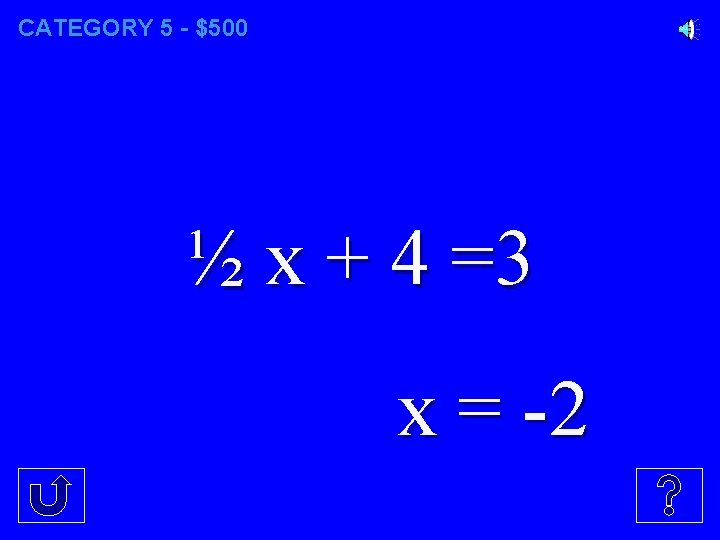CATEGORY 5 - \$500 ½ x + 4 =3 x = -2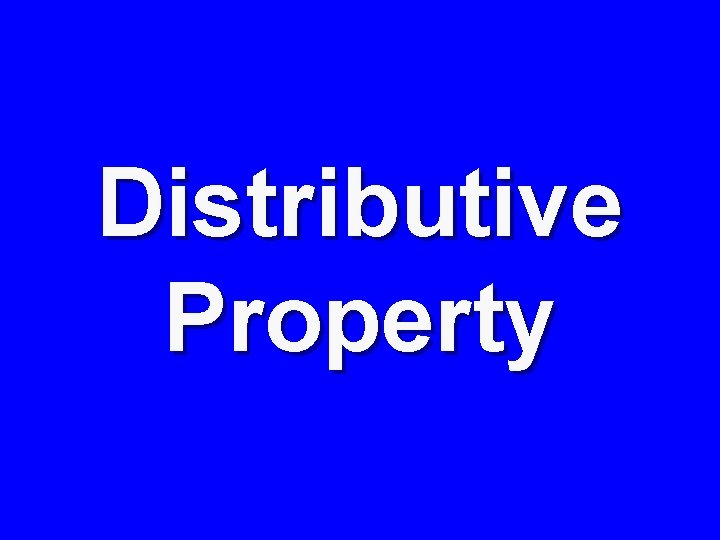Distributive PropertyFINAL CATEGORY Solve: ½ (x + 4) = -5 x = -14